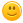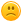+ Нов въпросnd_nikolov 7 Точки

## Help for 4.Social Distribution from Lists Advanced - More Exercises.

```Здравейте, ако някой е получил 100/100 на тази задача, би ли споделил с мен как го е постигнал. Опитах какво ли не, но явно пропускам нещо съществено защото две проверки не минават (60/100)
Ето и условието:```

A core idea of several left-wing ideologies is that the wealthiest should support the poorest, no matter what and that is exactly what you are called to do for this problem.

On the first line you will be given the population (numbers separated by comma and space ", "). On the second line you will be given the minimum wealth. You have to distribute the wealth, so that there is no part of the population that has less than the minimum wealth. To do that, you should always take wealth from the wealthiest part of the population. There will be cases, where the distribution will not be possible. In that case, print "No equal distribution possible"

Example:

 Input Output 2, 3, 5, 15, 75 5 [5, 5, 5, 15, 70] 2, 3, 5, 15, 75 20 [20, 20, 20, 20, 20] 2, 3, 5, 45, 45 30 No equal distribution possible
```
population = input().split(', ')
wealth_level = int(input())
population_list = sorted(list(map(lambda x: int(x), population)))
max_element = max(population_list)
min_element = min(population_list)
length_population_list = len(population_list)
low_element = 0

if sum(population_list) / length_population_list < wealth_level or wealth_level == 0 or min_element >= wealth_level:
print('No equal distribution possible')

else:
for element in population_list:
if element <= wealth_level:
min_element = element
population_list.remove(element)
population_list.insert(0, wealth_level)
population_list.remove(max_element)
max_element -= (wealth_level - min_element)
population_list.append(max_element)
else:
element = element

max_element = max(population_list)

print(population_list)```
Тагове:
1niyazihasan 82 Точки

Здравейте,

Ето примерно 100/100 решение, дано да Ви помогне да откриете какво пропускате.

numbers = [int(num) for num in input().split(", ")]
minimum_wealth = int(input())

for i in range(len(numbers)):
if numbers[i] < minimum_wealth:
c = minimum_wealth - numbers[i]
max_number = max(numbers)
if max_number - c >= minimum_wealth:
numbers[numbers.index(max_number)] -= c
numbers[i] += c
if sum(numbers) >= len(numbers) * minimum_wealth:
print(numbers)
else:
print("No equal distribution possible")

1
30/06/2020 22:51:31nd_nikolov 7 Точки

Благодаря!

1MartinBG 4761 Точки

Условието на задачата не е достатъчно конкретно, но ако приложим малко дедукция и логика ще стигнем до решение, подобно на това, което @nd_nikolov е дал:

- ако общото богатство е по-малко от брой хора * мин. богатство - No equal distribution possible

- винаги се взема от най-богатия (колкото трябва, без да се пада под мин. богатство) и се дава на най-бедния

- това се прави, докато всички имат поне мин. богатство

- изходът е сортиран по големина (предполага се от примерните тестове, но там и входът е сортиран)

Ако приложим горната логика, следните входни данни:

2, 6, 6, 6

5

Трябва да дадат резултат [5, 5, 5, 5]

Решението на @nd_nikolov ще изведе:

[5, 5, 6, 4]

Което очевидно е грешно

Решението на @niyazihasan ще изведе:

[2, 6, 6, 6]

Това е решението, което дава 100/100 в Judge, т.е. има вероятност и авторовото решение, използвано за генериране на двойките вход -> изход в Judge също да е имало подобен бъг... Или просто в Judge няма тест като този.При всички случаи, не си струва да се мъчите с изкарване на 100/100 в Judge за задачи, които са зле описани или има вероятност за грешно авторово решение (и съответно грешни отговори в системата).

Лошо е, когато това се случи с изпитна задача.Иначе, ето едно решение, което с горния вход ще произведе коректен резултат, т.е. [5, 5, 5, 5]

``````population = [int(num) for num in input().split(", ")]
wealth_level = int(input())

if sum(population) < len(population) * wealth_level:
print('No equal distribution possible')
else:
population.sort()
poorest_index = 0
richest_index = len(population) - 1
while population[poorest_index] < wealth_level:
needed = wealth_level - population[poorest_index]
can_take = population[richest_index] - wealth_level
redistributed_wealth = min(needed, can_take)
population[poorest_index] += redistributed_wealth
population[richest_index] -= redistributed_wealth
population.sort()

print(population)``````

EDIT:

Стигнах да следното работещо решение (както в Judge, така и с горните примерни данни), като грешката беше в допускането, че резултата е сортиран по големина (т.е. не трябва да го сортираме):

``````population = [int(num) for num in input().split(", ")]
wealth_level = int(input())

if sum(population) < len(population) * wealth_level:
print('No equal distribution possible')
else:
poorest = min(population)

while poorest < wealth_level:
poorest_index = population.index(poorest)
richest = max(population)
richest_index = population.index(richest)

needed = wealth_level - poorest
can_take = richest - wealth_level
redistributed_wealth = min(needed, can_take)
population[poorest_index] += redistributed_wealth
population[richest_index] -= redistributed_wealth

poorest = min(population)

print(population)
``````

0
01/07/2020 02:12:44nd_nikolov 7 Точки

Благодаря за коментарите и добрия съвет!

Ще го имам предвид на изпит.

1Julianh12 3 Точки

Ето ви едно просто и късо решение,задачата ми отне около 15 мин,попринцип някой ги решавам с дни,тука даже бях се отчаял предварително тъй като преди седмица гледах решенията ви и нищо не разбрах като цяло,но пък се радвам,че сам си измислил логиката,дори е вярна и при специални входове като 2,6,6,6:

```population=[int(x) for x in input().split(", ")]
minimum_wealth=int(input())
for i in range(len(population)) :
wealthiest=max(population)
poorest=min(population)
take_wealth=population.index(wealthiest)
give_poorest=population.index(poorest)
population[give_poorest]+=minimum_wealth-poorest
population[take_wealth]-=minimum_wealth-poorest

if min(population)>=minimum_wealth:
print(population)
else :
print("No equal distribution possible")

```
0BasilioCristov 0 Точки

Благодаря на всички колеги за тези решенията по-горе. Дебъгнах ги всички, но решението с което реших да остана,  и ми помогна много да разбера задачата е на "Julianh12". Позволих си да рефакторирам неговия код един "break" и "continue", с цел да се избегне обикаляне през листа ако това не е необходимо вече. Слагам рефактурирания код.

```population=[int(x) for x in input().split(", ")]
min_limit=int(input())
for i in range(len(population)) :
wealthiest=max(population)
if wealthiest <= min_limit:
break
poorest=min(population)
take_inx_rich=population.index(wealthiest)
take_inx_poor=population.index(poorest)
difer = min_limit - poorest
if difer == 0:
continue
population[take_inx_poor]+= difer  # Suma la diferencia al index mas pobre

population[take_inx_rich]-= difer  # Resta la diferencia al index mas rico

if not min(population)>= min_limit:  # Si actual pobreza es mayor o igual al min limit entra
print("No equal distribution possible")
else :
print(population)```
0
09/12/2021 13:22:01
Можем ли да използваме бисквитки?
Ние използваме бисквитки и подобни технологии, за да предоставим нашите услуги. Можете да се съгласите с всички или част от тях.
Назад
Функционални
Използваме бисквитки и подобни технологии, за да предоставим нашите услуги. Използваме „сесийни“ бисквитки, за да Ви идентифицираме временно. Те се пазят само по време на активната употреба на услугите ни. След излизане от приложението, затваряне на браузъра или мобилното устройство, данните се трият. Използваме бисквитки, за да предоставим опцията „Запомни Ме“, която Ви позволява да използвате нашите услуги без да предоставяте потребителско име и парола. Допълнително е възможно да използваме бисквитки за да съхраняваме различни малки настройки, като избор на езика, позиции на менюта и персонализирано съдържание. Използваме бисквитки и за измерване на маркетинговите ни усилия.
Рекламни
Използваме бисквитки, за да измерваме маркетинг ефективността ни, броене на посещения, както и за проследяването дали дадено електронно писмо е било отворено.